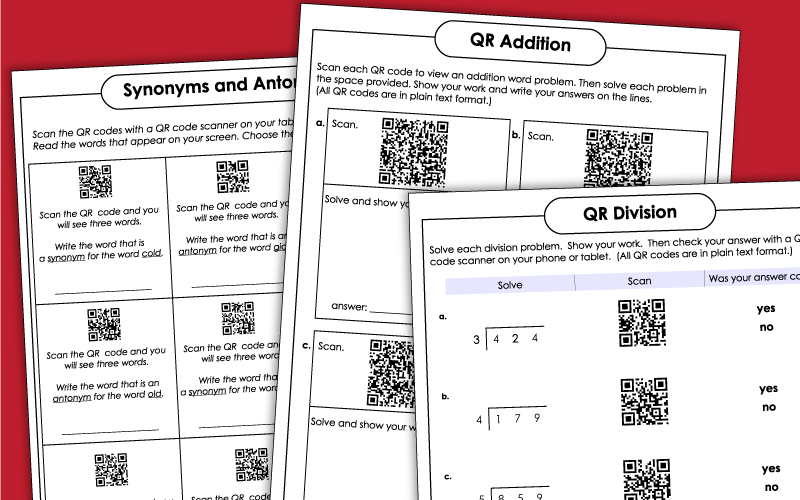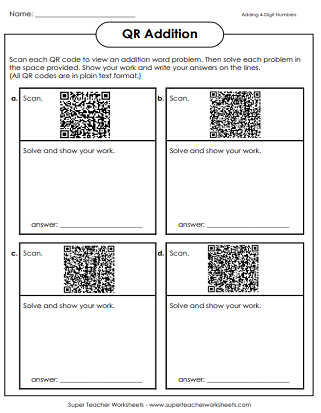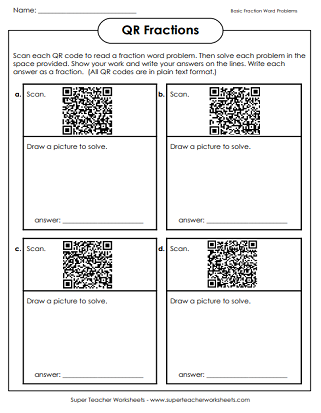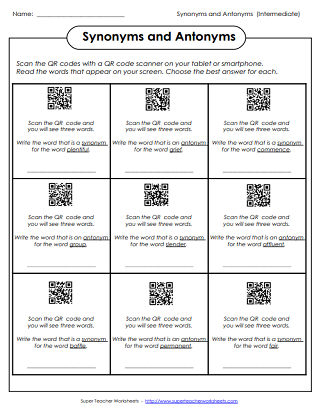# QR Code Worksheets

On these printable lessons, students can solve math problems and check their answers using a QR code scanner. Some worksheets will reveal a word problem with a when students scan a barcode.

Note: All of the activities on this page require students to use an iPad, iPhone, Android, or other device with a QR scanning app.Scan the QR code with an iPad or smartphone to view an addition word problem. Then find the sum of the 2-digit numbers. Show your work.
This printable includes 30 task cards with addition problems. Each problem has 2-digit addends. There's also an answer paper and a sheet that kids can use to show their work. Students can scan the QR code to check answers.
Use the QR scanner app on your tablet or phone to scan the barcode. A word problem will appear on your screen. Solve by finding the sum of the 3-digit numbers.
This file includes 30 task cards, each of which has a QR barcode on it. Student solve the 3-digit addition problems, then scan to check their answers. Includes a work page for students to solve problems.
This worksheet contains four QR codes. Students scan each code to reveal a word problem. They solve the problem in the space provided on the worksheet.
This file has 30 printable task cards. Student solve the 3-digit addition problem on each card. They can then scan the QR code to check their sums.
After scanning each barcode on this worksheet, students will be presented with an addition word problem. Solve the problems and show their work in the space provided.

## Subtraction

Scan the QR code with an iPad or smartphone to see a subtraction word problem. Solve the problem in the space provided. All subtraction problems have 2-digit numbers and require students to regroup.
This file has 30 printable task cards. Student solve the 2-digit subtraction problem on each card. (Most problems require regrouping/borrowing.) They then scan the QR code to check their answers.
To view each subtraction problem, students scan the given QR code. The subtraction problems on this worksheet have 3-digit numbers and require students to borrow.
This set includes 30 task cards. Students find the difference for each subtraction problem, then check by scanning the QR code with a tablet or phone.
This QR scan worksheet has word problems with 4-digit numbers that students must subtract.
Students solve to find the answers to the 4-digit subtraction problems on the task cards. Then check by scanning the barcode.
Scan the QR codes to see problems that require students to make change. All of these problems require students to borrow across zero.

## Multiplication

First multiply to find the product for each 2-digit by 1-digit math problem. Then scan the QR code with an iPad or smartphone to check your answer.
To view each word problem, students scan the QR code shown. Then they solve each problem and show their work. These problems require students to multiply 3-digit numbers by 1-digit numbers.
Students first calculate the product for each 3-digit by 1-digit multiplication problem. After that, they scan the QR code with an tablet or phone to check their answers.
For this activity, students multiply a money amount by a single-digit number. They check their answers by scanning the barcode shown.
Scan the code to view a 3-digit by 2-digit multiplication word problem. Then solve and show your work.
Solve each 3-digit by 2-digit multiplication problem and show your work. Then scan the QR code with an tablet or smartphone to check your answer.

## Division

Write the answers to the basic division problems. Scan to check. The problems on this worksheets are basic facts with divisors up to nine. (example: 72 divided by 8 equals 9)
This page has four basic division word problems. Scan to see the text of the word problem. Then solve in the space provided.
Solve the long division problems. Then scan the QR to check the answers. All division problems on this page have a 3-digit dividend and a 2 or 3-digit quotient.

## Fractions

Scan the barcode to reveal basic fraction word problems. Draw a picture to solve.

## ELA

Can you find the synonym or antonym for each word given? Scan the barcode and three words will appear. Choose the best answer and write it on the line. This version uses basic vocabulary for 2nd - 4th graders.
Can you find the synonym or antonym for each word given? Scan the barcode and three words will appear. Choose the best answer and write it on the line. This version uses more advanced vocabulary for 4th-6th graders.

Multiply 2, 3, and 4-digit numbers with these printable worksheets.

## Sample Worksheet ImagesMy Account
Site Information